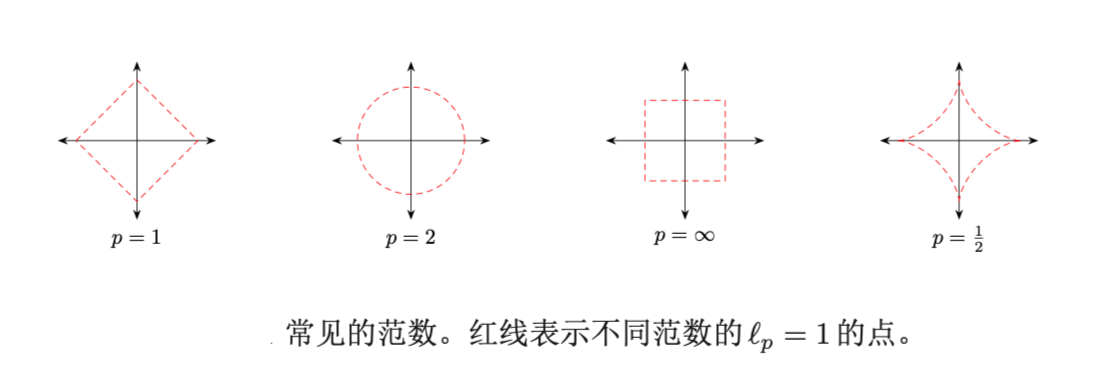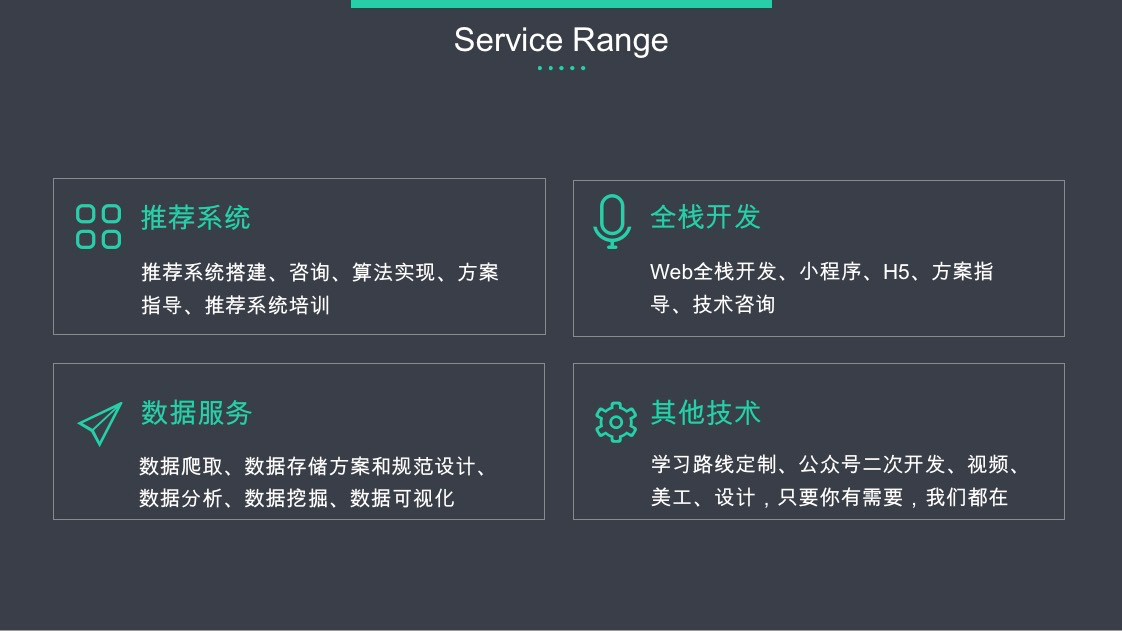【算法工程师的数学基础】系列将会从线性代数、微积分、数值优化、概率论、信息论五个方面进行介绍，感兴趣的欢迎关注【搜索与推荐Wiki】公众号，获得最新文章。

《算法工程师的数学基础》已更新：

## 向量和向量空间

### 向量空间

• 向量加法+：向量空间$V$中的两个向量$a、b$，他们的和$a+b$也属于向量空间
• 标量乘法$\cdot$：向量空间$V$中的任一向量$a$和任一标量$c$，他们的乘积$c \cdot a$也属于向量空间

• 含有零向量的向量组，必定线性相关。若有向量组$a_{1},a_{2},…,a_{s}$，其中$a_{1}=0$，则$a_{1}=0\cdot a_{2}+…+0\cdot a_{s}$。
• 含有两个相等向量的向量组，必定线性相关。若有向量组$a_{1},a_{2},…,a_{s}$，其中$a_{1}=a_{2}$，则$a_{1}=1\cdot a_{2}+0\cdot a_{3}+…+0\cdot a_{s}$。
• 若一向量组相关，则加上任意个向量后，仍然线性相关；即局部线性相关，整体必线性相关。
• 整体线性无关，局部必线性无关。
• 向量个数大于向量维数，则此向量组线性相关。
• 若一向量组线性无关，即使每一向量都在同一位置处增加一分量，仍然线性无关。
• 若一向量组线性相关，即使每一向量都在同一位置处减去一分量，仍然线性相关。
• 若$a_{1},a_{2},…,a_{s}$线性无关，而$b,a_{1},a_{2},…,a_{s}$线性相关，则$b$，b必可由$a_{1},a_{2},…,a_{s}$线性表示，且表示系数唯一。
• 有向量组${\textrm {I}}\{a_{1},a_{2},…,a_{s}\}$和${\textrm {II}}\{b_{1},b_{2},…,b_{t}\}$，其中$t>s$，且${\textrm {II}}$中每个向量都可由${\textrm {I}}$线性表示，则向量组${\textrm {II}}$必线性相关。即向量个数多的向量组，若可被向量个数少的向量组线性表示，则向量个数多的向量组必线性相关。
• 若一向量组$b_{1},b_{2},…,b_{t}$可由向量组$a_{1},a_{2},…,a_{s}$线性表示，且$b_{1},b_{2},…,b_{t}$线性无关，则$t\leq s$。即线性无关的向量组，无法以向量个数较少的向量组线性表示。

$n$维空间$V$的一组标准基（standard basis） 为：

$V$中的任一向量$v=[v_1, v_2, v_3, .., v_n]$可以唯一表示为：

$v_1, v_2, v_3, .., v_n$ 也称为向量$v$的笛卡尔坐标（Carterian coordinates）。

### 范数

$l_1$范数 为向量各个元素的绝对值之和。

$l_2$范数 为向量各个元素的平方和再开方

$l_2$范数又称为Euclidean范数或者Frobenius。从几何角度，向量也可以表示为从原点出发的一个带箭头的有向线段，其$l_2$范数为线段的长度，也常称为向量的模。

$l_{\infty}$范数 为向量各个元素的最大绝对值。### 常见的向量

one-hot向量 为有且已有一个元素为1，其余元素都为0的向量。

【技术服务】，详情点击查看： https://mp.weixin.qq.com/s/PtX9ukKRBmazAWARprGIAg